Processing ......FreeComputerBooks.com Links to Free Computer, Mathematics, Technical Books all over the World

Algorithms for Decision Making
🌠 Top Free Data Science Books - 100% Free or Open Source!
• Title Algorithms for Decision Making
• Author(s) Mykel Kochenderfer, Tim Wheeler, and Kyle Wray
• Publisher: The MIT Press (August 2, 2022); eBook (Creative Commons Edition)
• Hardcover: 704 pages
• eBook PDF (700 pages)
• Language: English
• ISBN-10: 0262047012
• ISBN-13: 978-0262047012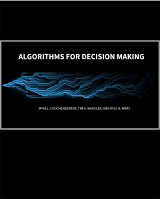Book Description

This book provides a broad introduction to algorithms for decision making under uncertainty. It covers a wide variety of topics related to decision making, introducing the underlying mathematical problem formulations and the algorithms for solving them. Figures, examples, and exercises are provided to convey the intuition behind the various approaches.

It requires some mathematical maturity and assumes prior exposure to multivariable calculus, linear algebra, and probability concepts. Some review material is provided in the appendices.

Disciplines where the book would be especially useful include mathematics, statistics, computer science, aerospace, electrical engineering, and operations research.

Fundamental to this textbook are the algorithms, which are all implemented in the Julia programming language.

• N/A
Reviews, Rating, and Recommendation: Related Book Categories: Read and Download Links:Similar Books:
•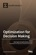Optimization for Decision Making: Linear and Quadratic Models

This book illustrates how to formulate real world problems using linear and quadratic models; It focus on developing modeling skills to support valid decision making for complex real world problems.

•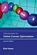Introduction to Online Convex Optimization (Elad Hazan)

This book presents a robust machine learning approach that contains elements of mathematical optimization, game theory, and learning theory: an optimization method that learns from experience as more aspects of the problem are observed.

•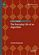The Everyday Life of an Algorithm (Daniel Neyland)

Through investigating the everyday life of the algorithm, the book opens a conversation with existing social science research that tends to focus on the power and opacity of algorithms, via unique access to the algorithm's design, development, testing, etc.

•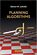Planning Algorithms (Steven M. LaValle)

This is the only book for teaching and referencing of Planning Algorithms in applications including robotics, computational biology, computer graphics, manufacturing, aerospace applications and medicine, etc.

•The Design of Approximation Algorithms (D. P. Williamson)

This book shows how to design approximation algorithms: efficient algorithms that find provably near-optimal solutions. is organized around central algorithmic techniques for designing approximation algorithms, including greedy and local search algorithms, dynamic programming, linear and semidefinite programming, and randomization.

•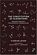The Constitution of Algorithms (Florian Jaton)

A provocative and skillful study of how algorithms come into the world - and inevitably shape it. Jaton performs the daring feat of offering an empirically rich analysis of algorithms without taking them for granted.

•Numerical Algorithms: Computer Vision, Machine Learning, etc.

This book presents a new approach to numerical analysis for modern computer scientists, covers a wide range of topics - from numerical linear algebra to optimization and differential equations - focusing on real-world motivation and unifying themes.

•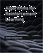Algorithms for Reinforcement Learning (Csaba Szepesvari)

This book focuses on those algorithms of reinforcement learning that build on the powerful theory of dynamic programming. It gives a fairly comprehensive catalog of learning problems, describe the core ideas, note a large number of state of the art algorithms.

•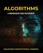Algorithms: Fundamental Techniques (Macneil Shonle, et al)

The goal of the book is to show you how you can methodically apply different techniques to your own algorithms to make them more efficient. While this book mostly highlights general techniques, some well-known algorithms are also looked at in depth.

•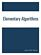Elementary Algorithms (Xinyu Liu)

This book doesn't only focus on an imperative (or procedural) approach, but also includes purely functional algorithms and data structures. It teaches you how to think like a programmer - find the practical efficiency algorithms to solve your problems.

•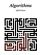Lecture Notes for the Algorithms (Jeff Erickson)

This lecture notes uniquely combines rigor and comprehensiveness. It covers a broad range of algorithms in depth, yet makes their design and analysis accessible to all levels of readers. Each chapter is relatively self-contained and can be used as a unit of study.

•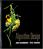Algorithm Design (Jon Kleinberg, et al)

This book introduces algorithms by looking at the real-world problems that motivate them. The book teaches a range of design and analysis techniques for problems that arise in computing applications.

Book Categories
 :All CategoriesTop Free BooksRecent BooksMiscellaneous BooksComputer EngineeringComputer LanguagesComputer ScienceData Science/DatabasesJava and Java EE (J2EE)Linux and UnixMathematicsMicrosoft and .NETMobile ComputingNetworking and CommunicationsSoftware EngineeringSpecial TopicsWeb Programming
Other Categories